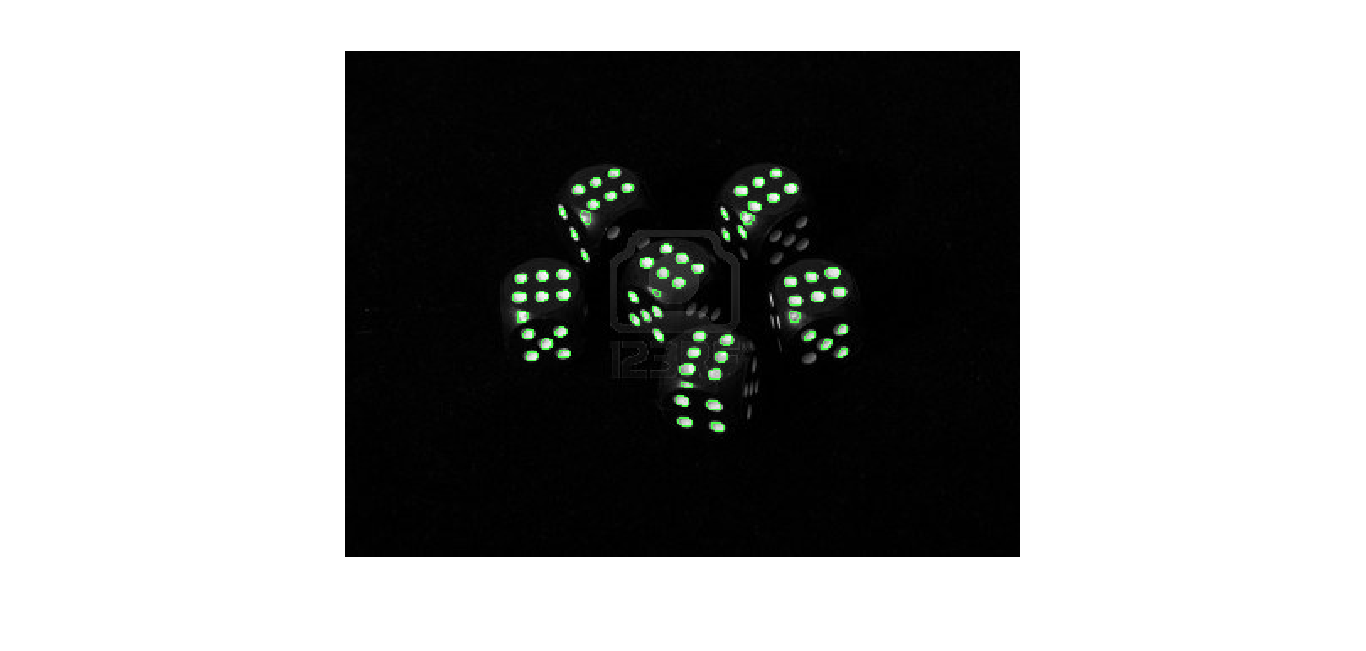Browse By

Many a times, we need to count certain number of objects in an image. The purpose might be for a traffic analysis from the video stream that is coming in, or to analyse how many cells are present in the image taken from a microscope. Sometimes, we are just trying to learn some image processing functions in MATLAB and we are stuck at the point where we need to count the number of objects present in a given picture.

Getting started with reading an image

The most important step while writing any kind of program for image processing is, you need to read the image file and store it in a data base.Now, there can be images which are RGB. But all our processing takes place in black and white format. So we need to convert it into gray scale using a simple line of code.

Images are usually in truecolour format as mentioned before, which is RGB. RGB images contain 2 types of visual information, one contains the chrominance, that's the hue and saturation information and the other type of information is the luminance.

The next step for us is to convert the entire grayscale image to a binary image. A binary image contains only 2 values, white and black, unlike the grayscale image which contains a mixture of black and white.

The function im2bw(I, level); contains two arguments, one is the image and the other one is the level of threshold. Any pixel which has a value greater that the threshold is converted into a white pixel, while the pixels below threshold are blackened out. Threshold level is calculated using the function graythreshold(I) which takes the image as an argument.

Now we need to find a bunch of pixels which would not be seen as a part of the object we are trying to detect. A few random white spots inside the object might misguide the algorithm to consider them as separate object. The imfill() function will fill the areas of image which we define.

Check out the example from the Matlab referenceUnwanted black pixels inside the white circles are converted into white pixels which can be seen by the computer as a single object. In some cases, the image might not be up to the mark for further processing. In that case, we needed to use the imopen() function which morphologically fills the image. Check out the screenshot of the result.

Now, we can clearly see that all the light parts of the image are seperated into individual bunch of white pixels which are connected to each other. Now, all we need to do is to plot a perimeter across them to visually see how we have been able to detect the object in the image.

The function bwareaopen() is used to remove any small blobs of white which are very close to each other. Once that is done, we plot a perimeter around all the white blobs and then overlay the perimeter on the actual image. Once we plot the perimeter, all we got to do is the count the number of regions encircled by our perimeter function. This is done simply by using a function called bwconncomp();

bwnconncomp() returns a structure which is stored in CC. The structure has four fields: 1. Connectivity 2. Size of the image 3. Number of objects and 4. 1-by-NumObjects cell array where the kth element in the cell array is a vector containing the linear indices of the pixels in the kth object.

By accessing the CC.numObjects field of the structure, we get the information of how many objects have been detected in the image.The white spots on the dice get detected. They are encircled in green using the matlab function bwperim().

This program written in Matlab is a very very basic program for detecting simple white objects in a black and white image. But, this is the first step into learning the more complex algorithms used for tracking moving objects, recognizing faces and objects in a video frame, and many many more interesting things. Hope you enjoyed this very basic tutorial on object counting using the image processing toolbox of Matlab.